Science, Maths & Technology

### Become an OU studentEveryday maths 1

Start this free course now. Just create an account and sign in. Enrol and complete the course for a free statement of participation or digital badge if available.

# 3 Estimating, measuring and comparing weights

How much do you weigh?

You might have given your weight in kilograms (kg) or in pounds (lb), or pounds and stone (st). Kilograms are metric weights. Pounds and stones are imperial weights.

• 1,000 grams (g) = 1 kilogram (kg)

You should measure weight in metric units, but you might see the old imperial units used sometimes.

• 1 g is approximately the weight of a paperclip.

Many foods are sold by weight. For example:

• 1 kg of sugar (this is equivalent to about two and a quarter pounds)
• 500 g of rice (about one pound)
• 250 g of coffee (about half a pound)
• 100 g of chocolate (slightly less than a quarter of a pound)
• 30 g of crisps (about an ounce)
• 10 g of a spice (about a third of an ounce).

Heavier things are weighed in kilograms:

• 50 kg of sharp sand (about 110 pounds)
• 10 kg chicken food (about 20 pounds)
• 1 kg of nails (about two pounds).

Note that if you bought ten packets of rice, you would say you had bought 5 kg rather than 5,000 g.

Scales show you how much something weighs. Digital scales show the weight as a display of numbers. Other scales have a dial or line of numbers and you have to read the weight from this.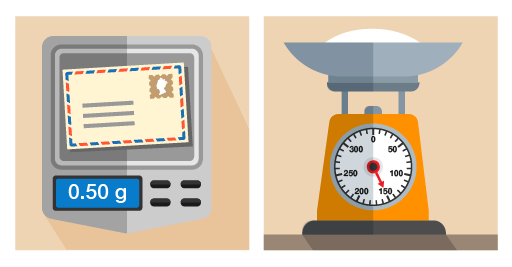Figure 22 Using different scales for different objects

You’ll notice that on the right-hand set of scales in the picture above, the needle points to 150 g. If you use scales like this, you need to know the divisions marked on the scales. You might have to count the marks between numbers.

## Example: Identifying weights on scales

What is the weight of the flour in these scales?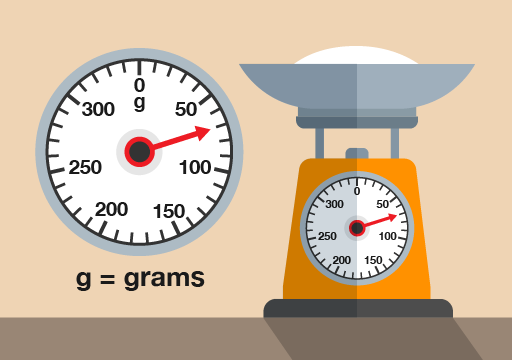Figure 23 Weighing flour

(Note that scales like this are calibrated to weigh only the flour inside the bowl – the weight on the scales is just the flour, not the flour and the bowl.)

### Method

There are four marks between 50 g and 100 g, each representing another 10 g. So the marks represent 60 g, 70 g, 80 g and 90 g. The needle is level with the second mark, so the weight is 70 g.

Now try the following activity. Remember to check your answers once you have completed the questions.

1. How many grams of sugar are on the scales in the picture below?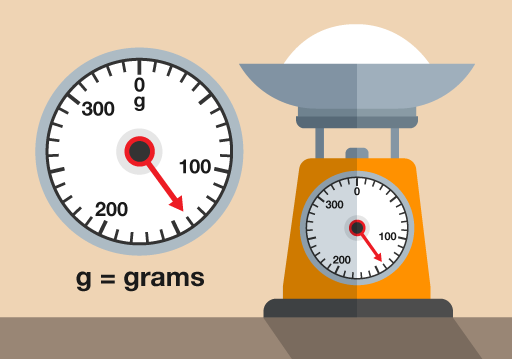Figure 24 Weighing sugar
2. What is this person’s weight in kilograms?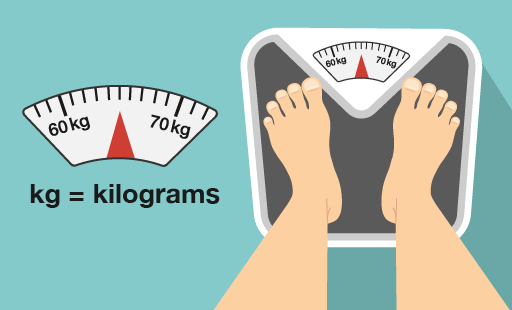Figure 25 Weighing a person
3. How much does the letter weigh?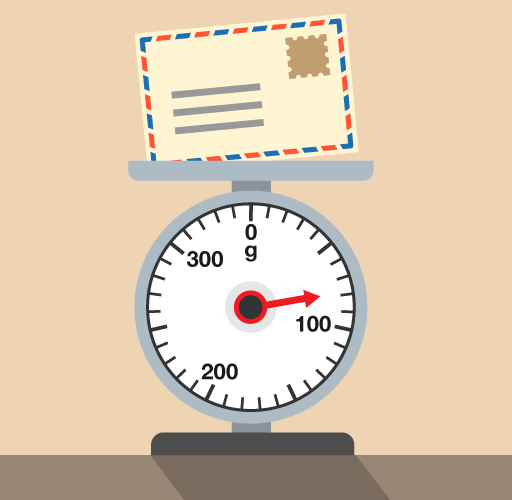Figure 26 Weighing a letter